Publicité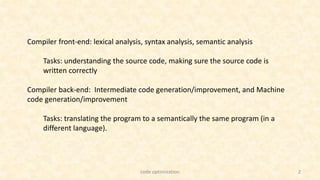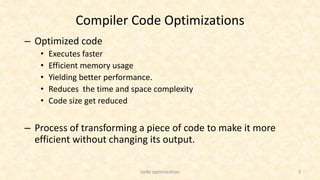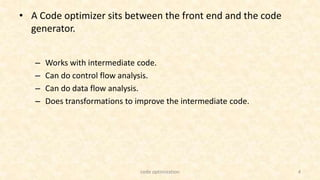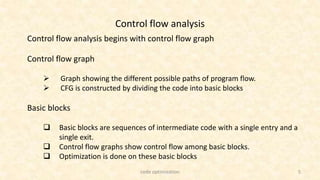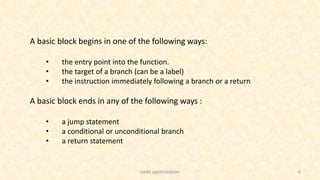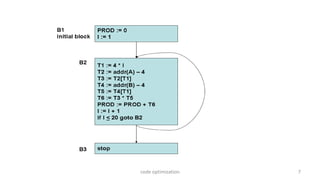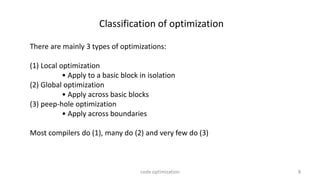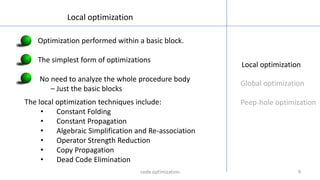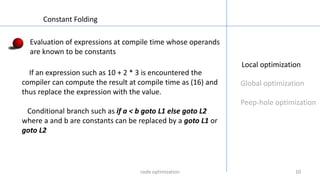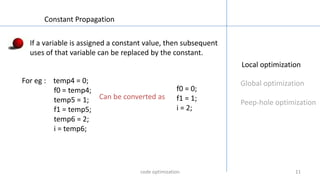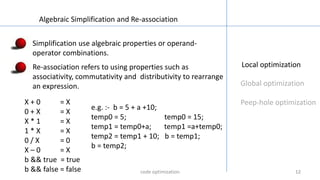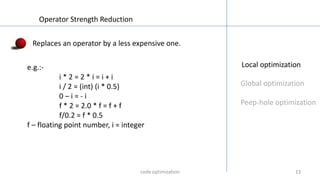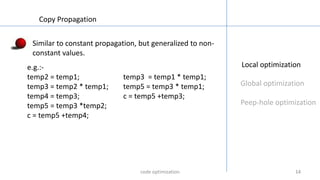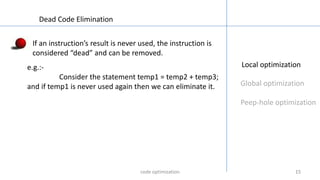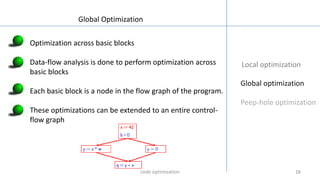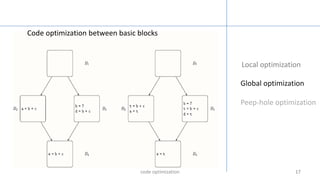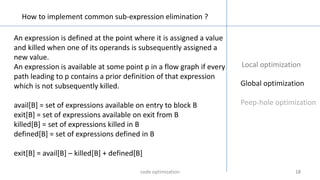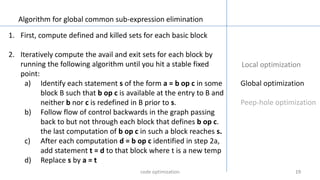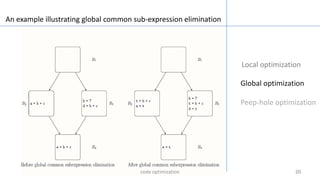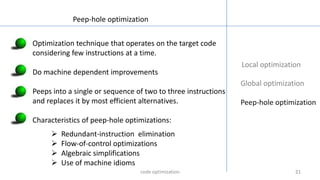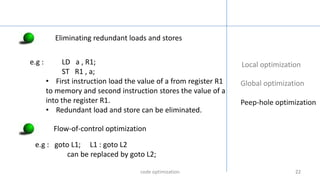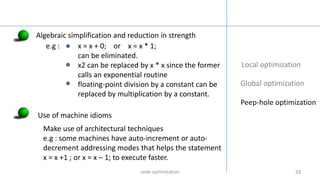1 sur 24
Publicité

### Code optimization

1. 1 Veena venugopal COS 140512 code optimization
2. 2 Compiler front-end: lexical analysis, syntax analysis, semantic analysis Tasks: understanding the source code, making sure the source code is written correctly Compiler back-end: Intermediate code generation/improvement, and Machine code generation/improvement Tasks: translating the program to a semantically the same program (in a different language). code optimization
3. 3 Compiler Code Optimizations – Optimized code • Executes faster • Efficient memory usage • Yielding better performance. • Reduces the time and space complexity • Code size get reduced – Process of transforming a piece of code to make it more efficient without changing its output. code optimization
4. 4 • A Code optimizer sits between the front end and the code generator. – Works with intermediate code. – Can do control flow analysis. – Can do data flow analysis. – Does transformations to improve the intermediate code. code optimization
5. 5 Control flow analysis Control flow analysis begins with control flow graph Control flow graph  Graph showing the different possible paths of program flow.  CFG is constructed by dividing the code into basic blocks Basic blocks  Basic blocks are sequences of intermediate code with a single entry and a single exit.  Control flow graphs show control flow among basic blocks.  Optimization is done on these basic blocks code optimization
6. 6 A basic block begins in one of the following ways: • the entry point into the function. • the target of a branch (can be a label) • the instruction immediately following a branch or a return A basic block ends in any of the following ways : • a jump statement • a conditional or unconditional branch • a return statement code optimization
7. 7code optimization
8. 8 Classification of optimization There are mainly 3 types of optimizations: (1) Local optimization • Apply to a basic block in isolation (2) Global optimization • Apply across basic blocks (3) peep-hole optimization • Apply across boundaries Most compilers do (1), many do (2) and very few do (3) code optimization
9. 9 Local optimization Global optimization Peep-hole optimization Local optimization Optimization performed within a basic block. The simplest form of optimizations No need to analyze the whole procedure body – Just the basic blocks The local optimization techniques include: • Constant Folding • Constant Propagation • Algebraic Simplification and Re-association • Operator Strength Reduction • Copy Propagation • Dead Code Elimination code optimization
10. 1010 Local optimization Global optimization Peep-hole optimization Constant Folding Evaluation of expressions at compile time whose operands are known to be constants If an expression such as 10 + 2 * 3 is encountered the compiler can compute the result at compile time as (16) and thus replace the expression with the value. Conditional branch such as if a < b goto L1 else goto L2 where a and b are constants can be replaced by a goto L1 or goto L2 code optimization
11. 1111 Local optimization Global optimization Peep-hole optimization Constant Propagation If a variable is assigned a constant value, then subsequent uses of that variable can be replaced by the constant. For eg : temp4 = 0; f0 = temp4; temp5 = 1; f1 = temp5; temp6 = 2; i = temp6; f0 = 0; f1 = 1; i = 2; Can be converted as code optimization
12. 1212 Local optimization Global optimization Peep-hole optimization Algebraic Simplification and Re-association Simplification use algebraic properties or operand- operator combinations. Re-association refers to using properties such as associativity, commutativity and distributivity to rearrange an expression. X + 0 = X 0 + X = X X * 1 = X 1 * X = X 0 / X = 0 X – 0 = X b && true = true b && false = false e.g. :- b = 5 + a +10; temp0 = 5; temp0 = 15; temp1 = temp0+a; temp1 =a+temp0; temp2 = temp1 + 10; b = temp1; b = temp2; code optimization
13. 1313 Local optimization Global optimization Peep-hole optimization Operator Strength Reduction Replaces an operator by a less expensive one. e.g.:- i * 2 = 2 * i = i + i i / 2 = (int) (i * 0.5) 0 – i = - i f * 2 = 2.0 * f = f + f f/0.2 = f * 0.5 f – floating point number, i = integer code optimization
14. 141414 Local optimization Global optimization Peep-hole optimization Copy Propagation Similar to constant propagation, but generalized to non- constant values. e.g.:- temp2 = temp1; temp3 = temp1 * temp1; temp3 = temp2 * temp1; temp5 = temp3 * temp1; temp4 = temp3; c = temp5 +temp3; temp5 = temp3 *temp2; c = temp5 +temp4; code optimization
15. 151515 Local optimization Global optimization Peep-hole optimization Dead Code Elimination If an instruction’s result is never used, the instruction is considered “dead” and can be removed. e.g.:- Consider the statement temp1 = temp2 + temp3; and if temp1 is never used again then we can eliminate it. code optimization
16. 161616 Local optimization Global optimization Peep-hole optimization Global Optimization Optimization across basic blocks Data-flow analysis is done to perform optimization across basic blocks Each basic block is a node in the flow graph of the program. These optimizations can be extended to an entire control- flow graph code optimization
17. 17171717 Local optimization Global optimization Peep-hole optimization Code optimization between basic blocks code optimization
18. 18181818 Local optimization Global optimization Peep-hole optimization How to implement common sub-expression elimination ? An expression is defined at the point where it is assigned a value and killed when one of its operands is subsequently assigned a new value. An expression is available at some point p in a flow graph if every path leading to p contains a prior definition of that expression which is not subsequently killed. avail[B] = set of expressions available on entry to block B exit[B] = set of expressions available on exit from B killed[B] = set of expressions killed in B defined[B] = set of expressions defined in B exit[B] = avail[B] – killed[B] + defined[B] code optimization
19. 19191919 Local optimization Global optimization Peep-hole optimization Algorithm for global common sub-expression elimination 1. First, compute defined and killed sets for each basic block 2. Iteratively compute the avail and exit sets for each block by running the following algorithm until you hit a stable fixed point: a) Identify each statement s of the form a = b op c in some block B such that b op c is available at the entry to B and neither b nor c is redefined in B prior to s. b) Follow flow of control backwards in the graph passing back to but not through each block that defines b op c. the last computation of b op c in such a block reaches s. c) After each computation d = b op c identified in step 2a, add statement t = d to that block where t is a new temp d) Replace s by a = t code optimization
20. 20202020 Local optimization Global optimization Peep-hole optimization An example illustrating global common sub-expression elimination code optimization
21. 21212121 Local optimization Global optimization Peep-hole optimization Peep-hole optimization Optimization technique that operates on the target code considering few instructions at a time. Do machine dependent improvements Peeps into a single or sequence of two to three instructions and replaces it by most efficient alternatives. Characteristics of peep-hole optimizations:  Redundant-instruction elimination  Flow-of-control optimizations  Algebraic simplifications  Use of machine idioms code optimization
22. 2222222222 Local optimization Global optimization Peep-hole optimization e.g : LD a , R1; ST R1 , a; • First instruction load the value of a from register R1 to memory and second instruction stores the value of a into the register R1. • Redundant load and store can be eliminated. Flow-of-control optimization Eliminating redundant loads and stores e.g : goto L1; L1 : goto L2 can be replaced by goto L2; code optimization
23. 232323232323 Local optimization Global optimization Peep-hole optimization Algebraic simplification and reduction in strength e.g : x = x + 0; or x = x * 1; can be eliminated. x2 can be replaced by x * x since the former calls an exponential routine floating-point division by a constant can be replaced by multiplication by a constant. Use of machine idioms Make use of architectural techniques e.g : some machines have auto-increment or auto- decrement addressing modes that helps the statement x = x +1 ; or x = x – 1; to execute faster. code optimization
24. code optimization 24 Thank you…..
Publicité